1. When the following are evaluated, how many of the answers are odd numbers?

A:B:C:D:E:2. The positive integer 2018 is the product of two primes.

What is the sum of these two primes?

A:B:C:D:E:3.

A: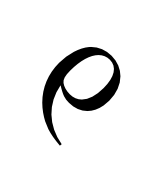B: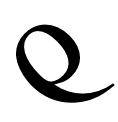C: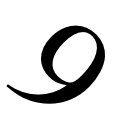D: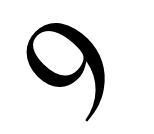E: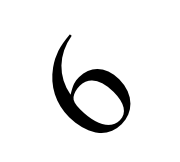4. Which of the following is not a multiple of 5?

A:B:C:D:E:5. Which of the following numbers is the largest?

A: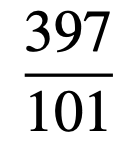B: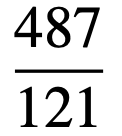C: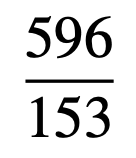D: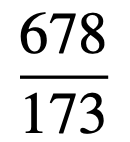E: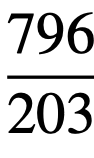6. Which of the following is equal to 25 × 15 × 9 × 5.4 × 3.24?

A:B:C:D:E:7

A: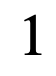B: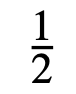C: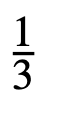D: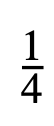E: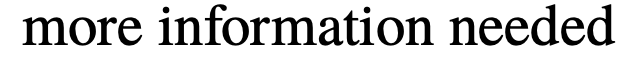8. What are the last two digits of 7²⁰¹⁸?

A:B:C:D:E:9. The diagram shows a rectangle AE F J inside a regular decagon ABCDEFGHIJ.

What is the ratio of the area of the rectangle to the area of the decagon?

A:B:C:D:E:10. On a training ride, Laura averages speeds of 12 km/h for 5 minutes, then 15 km/h for10 minutes and finally 18 km/h for 15 minutes.

What was her average speed over the whole ride?

A:B:C:D:E:11. How many of the following four equations has a graph that does not pass through the origin?

y = x ⁴ + 1       y = x⁴ + x     y = x⁴ + x²      y = x⁴ + x³

A:B:C:D:E:12. A regular tetrahedron is a polyhedron with four faces, each of which is an equilateral triangle, as shown. A solid regular tetrahedron is cut into two pieces by a single plane cut.

Which of the following could not be the shape of the section formed by the cut?

A:B:C:D:E:13. The lines y = x and y = mx − 4 intersect at the point P.

What is the sum of the positive integer values of m for which the coordinates of P are also positive integers?

A:B:C:D:E:14. The following twelve integers are written in ascending order:

1,x,x,x,y,y,y,y,y,8,9,11.

The mean of these twelve integers is 7. What is the median?

A:B:C:D:E:15.A square is inscribed in a circle of radius 1. An isosceles triangle is inscribed in the square as shown.

What is the ratio of the area of this triangle to the area of thE shaded region?

A: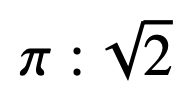B: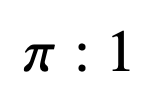C: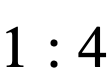D: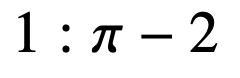E: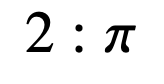Back to the top